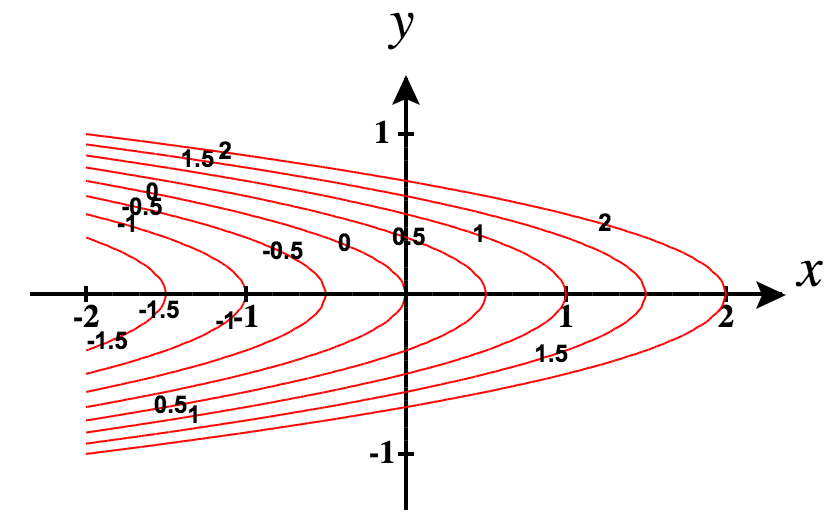$$\newcommand{\id}{\mathrm{id}}$$ $$\newcommand{\Span}{\mathrm{span}}$$ $$\newcommand{\kernel}{\mathrm{null}\,}$$ $$\newcommand{\range}{\mathrm{range}\,}$$ $$\newcommand{\RealPart}{\mathrm{Re}}$$ $$\newcommand{\ImaginaryPart}{\mathrm{Im}}$$ $$\newcommand{\Argument}{\mathrm{Arg}}$$ $$\newcommand{\norm}{\| #1 \|}$$ $$\newcommand{\inner}{\langle #1, #2 \rangle}$$ $$\newcommand{\Span}{\mathrm{span}}$$

# 14R: Chapter 14 Review Exercises

$$\newcommand{\vecs}{\overset { \rightharpoonup} {\mathbf{#1}} }$$ $$\newcommand{\vecd}{\overset{-\!-\!\rightharpoonup}{\vphantom{a}\smash {#1}}}$$$$\newcommand{\id}{\mathrm{id}}$$ $$\newcommand{\Span}{\mathrm{span}}$$ $$\newcommand{\kernel}{\mathrm{null}\,}$$ $$\newcommand{\range}{\mathrm{range}\,}$$ $$\newcommand{\RealPart}{\mathrm{Re}}$$ $$\newcommand{\ImaginaryPart}{\mathrm{Im}}$$ $$\newcommand{\Argument}{\mathrm{Arg}}$$ $$\newcommand{\norm}{\| #1 \|}$$ $$\newcommand{\inner}{\langle #1, #2 \rangle}$$ $$\newcommand{\Span}{\mathrm{span}}$$ $$\newcommand{\id}{\mathrm{id}}$$ $$\newcommand{\Span}{\mathrm{span}}$$ $$\newcommand{\kernel}{\mathrm{null}\,}$$ $$\newcommand{\range}{\mathrm{range}\,}$$ $$\newcommand{\RealPart}{\mathrm{Re}}$$ $$\newcommand{\ImaginaryPart}{\mathrm{Im}}$$ $$\newcommand{\Argument}{\mathrm{Arg}}$$ $$\newcommand{\norm}{\| #1 \|}$$ $$\newcommand{\inner}{\langle #1, #2 \rangle}$$ $$\newcommand{\Span}{\mathrm{span}}$$

For the following exercises, determine whether the statement is true or false. Justify your answer with a proof or a counterexample.

1.  The domain of $$f(x,y)=x^3\arcsin(y)$$ is  $$\big\{ (x,y) \, | \, x \in \mathbb R\text{ and }−\pi≤y≤\pi \big\}.$$

2.  If the function $$f(x,y)$$ is continuous everywhere, then $$f_{xy}(x,y) =f_{yx}(x,y).$$

True, by Clairaut’s theorem

3.  The linear approximation to the function of $$f(x,y)=5x^2+x\tan y$$ at the point $$(2,π)$$ is given by $$L(x,y)=22+21(x−2)+(y−π).$$

4.  $$(34,916)$$ is a critical point of $$g(x,y)=4x^3−2x^2y+y^2−2.$$

False

For the following exercises, sketch the function in one graph and, in a second, sketch several level curves.

5.  $$f(x,y)=e^{−\left(x^2+2y^2\right)}$$

6.  $$f(x,y)=x+4y^2$$For the following exercises, evaluate the following limits, if they exist. If they do not exist, prove it.

7.  $$\displaystyle \lim_{(x,y)→(1,1)}\frac{4xy}{x−2y^2}$$

8.  $$\displaystyle \lim_{(x,y)→(0,0)}\frac{4xy}{x−2y^2}$$

Does not exist.

For the following exercises, find the largest interval of continuity for the function.

9.  $$f(x,y)=x^3\arcsin y$$

10.  $$g(x,y)=\ln(4−x^2−y^2)$$

Continuous at all points on the $$xy$$-plane, except where $$x^2 + y^2 > 4.$$

For the following exercises, find all first partial derivatives.

11.  $$f(x,y)=x^2−y^2$$

12.  $$u(x,y)=x^4−3xy+1,$$ with $$x=2t$$ and $$y=t^3$$

$$\dfrac{∂u}{∂x}=4x^3−3y,$$

$$\dfrac{∂u}{∂y}=−3x,$$

$$\dfrac{dx}{dt} = 2$$  and $$\dfrac{dy}{dt} = 3t^2$$

\begin{align*} \dfrac{du}{dt} &= \dfrac{∂u}{∂x}\cdot\dfrac{dx}{dt} + \dfrac{∂u}{∂y}\cdot\dfrac{dy}{dt}\\[4pt] &= 8x^3 -6y -9xt^2\\[4pt] &= 8\big(2t\big)^3 - 6(t^3) - 9(2t)t^2 \\[4pt] &= 64t^3 - 6t^3 - 18t^3 \\[4pt] &= 40t^3 \end{align*}

For the following exercises, find all second partial derivatives.

13.  $$g(t,x)=3t^2−\sin(x+t)$$

14.  $$h(x,y,z)=\dfrac{x^3e^{2y}}{z}$$

$$h_{xx}(x,y,z) = \dfrac{6xe^{2y}}{z},$$
$$h_{xy}(x,y,z) = \dfrac{6x^2e^{2y}}{z},$$
$$h_{xz}(x,y,z) = −\dfrac{3x^2e^{2y}}{z^2},$$
$$h_{yx}(x,y,z) = \dfrac{6x^2e^{2y}}{z},$$
$$h_{yy}(x,y,z) = \dfrac{4x^3e^{2y}}{z},$$
$$h_{yz}(x,y,z) = −\dfrac{2x^3e^{2y}}{z^2},$$
$$h_{zx}(x,y,z) = −\dfrac{3x^2e^{2y}}{z^2},$$
$$h_{zy}(x,y,z) = −\dfrac{2x^3e^{2y}}{z^2},$$
$$h_{zz}(x,y,z) = \dfrac{2x^3e^{2y}}{z^3}$$

For the following exercises, find the equation of the tangent plane to the specified surface at the given point.

15.  $$z=x^3−2y^2+y−1$$ at point $$(1,1,−1)$$

16.  $$z=e^x+\dfrac{2}{y}$$ at point $$(0,1,3)$$

$$z = x - 2y + 5$$

17.  Approximate $$f(x,y)=e^{x^2}+\sqrt{y}$$ at $$(0.1,9.1).$$ Write down your linear approximation function $$L(x,y).$$ How accurate is the approximation to the exact answer, rounded to four digits?

18.  Find the differential $$dz$$ of $$h(x,y)=4x^2+2xy−3y$$ and approximate $$Δz$$ at the point $$(1,−2).$$ Let $$Δx=0.1$$ and $$Δy=0.01.$$

$$dz=4\,dx−dy, \; dz(0.1,0.01)=0.39, \; Δz = 0.432$$

19.  Find the directional derivative of $$f(x,y)=x^2+6xy−y^2$$ in the direction $$\vecs v=\mathbf{\hat i}+4\,\mathbf{\hat j}.$$

20.  Find the maximal directional derivative magnitude and direction for the function $$f(x,y)=x^3+2xy−\cos(πy)$$ at point $$(3,0).$$

$$3\sqrt{85}\langle 27, 6\rangle$$

For the following exercises, find the gradient.

21.  $$c(x,t)=e(t−x)^2+3\cos t$$

22.  $$f(x,y)=\dfrac{\sqrt{x}+y^2}{xy}$$

$$\vecs \nabla f(x, y) = -\dfrac{\sqrt{x}+2y^2}{2x^2y}\,\mathbf{\hat i} + \left( \dfrac{1}{x} + \dfrac{1}{\sqrt{x}y^2} \right) \,\mathbf{\hat j}$$

For the following exercise, find and classify the critical points.

23.  $$z=x^3−xy+y^2−1$$

For the following exercises, use Lagrange multipliers to find the maximum and minimum values for the functions with the given constraints.

24.  $$f(x,y)=x^2y,$$  subject to the constraint:  $$x^2+y^2=4$$

maximum: $$\dfrac{16}{3\sqrt{3}},$$ minimum: $$-\dfrac{16}{3\sqrt{3}},$$

25.  $$f(x,y)=x^2−y^2,$$  subject to the constraint:  $$x+6y=4$$

26.  A machinist is constructing a right circular cone out of a block of aluminum. The machine gives an error of $$5\%$$ in height and $$2\%$$ in radius. Find the maximum error in the volume of the cone if the machinist creates a cone of height $$6$$ cm and radius $$2$$ cm.

$$2.3228$$ cm3
27.  A trash compactor is in the shape of a cuboid. Assume the trash compactor is filled with incompressible liquid. The length and width are decreasing at rates of $$2$$ ft/sec and $$3$$ ft/sec, respectively. Find the rate at which the liquid level is rising when the length is $$14$$ ft, the width is $$10$$ ft, and the height is $$4$$ ft.